Courses

# Propagation Of EM Waves And Gauge Transformation MCQ Level - 1

## 10 Questions MCQ Test Topic wise Tests for IIT JAM Physics | Propagation Of EM Waves And Gauge Transformation MCQ Level - 1

Description
This mock test of Propagation Of EM Waves And Gauge Transformation MCQ Level - 1 for Physics helps you for every Physics entrance exam. This contains 10 Multiple Choice Questions for Physics Propagation Of EM Waves And Gauge Transformation MCQ Level - 1 (mcq) to study with solutions a complete question bank. The solved questions answers in this Propagation Of EM Waves And Gauge Transformation MCQ Level - 1 quiz give you a good mix of easy questions and tough questions. Physics students definitely take this Propagation Of EM Waves And Gauge Transformation MCQ Level - 1 exercise for a better result in the exam. You can find other Propagation Of EM Waves And Gauge Transformation MCQ Level - 1 extra questions, long questions & short questions for Physics on EduRev as well by searching above.
QUESTION: 1

### An electromagnetic wave is propagating in free space in the z-direction. If the electric field is given by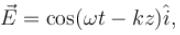where ωt = ck then the magnetic field is given by

Solution: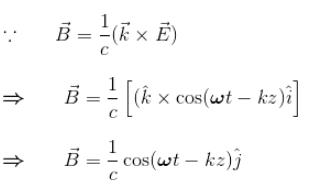The correct answer is: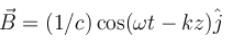QUESTION: 2

### The electric field for a plane electromagnetic wave traveling in the positive z-direction is represented by which one of the following? Where,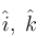are the unit vectors along x, z-directions respectively.

Solution:

The wave travelling along Z-axis can be written as, displacement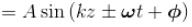+ sign along –ve Z-axis
– sign along +ve Z-axis
Since, the electric field is perpendicular to the different of the wave, i.e., perpendicular to Z-axis i.e., along X-axis or along Y-axis. Thus,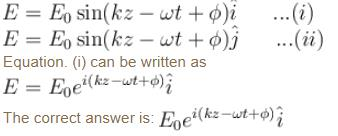QUESTION: 3

### In an electromagnetic wave, the direction of the magnetic induction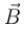is

Solution:

Electromagnetic waves are transverse in nature, i.e., both E and  B  are perpendicular to each other.

The correct answer is: perpendicular to the electric field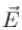QUESTION: 4

Imagine an electromagnetic wave in vacuum whose electric field (in SI units) is given by Ex(zt) = 102 sinπ(3 × 106z – 9 × 1014t), Ey = 0 and Ez = 0.
What is the magnetic field associated with the wave?

Solution:

Electric field is given as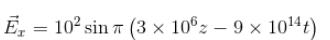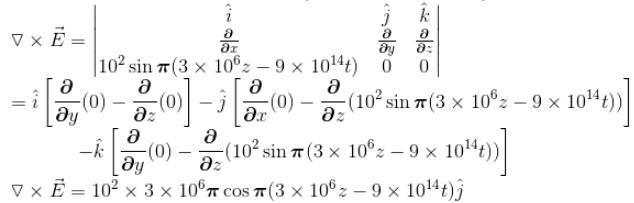Applying Maxwell Equation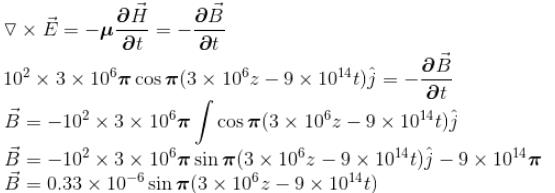The correct answer is: Bx(z, t) = 0.33 × 10–6sinπ(3 × 106z – 9 × 1014t), Bx = 0 and Bz = 0

QUESTION: 5

The direction of propagation of electromagnetic wave is given by

Solution:
QUESTION: 6

If a plane electromagnetic wave propagating in space has an electric field of amplitude 9 × 103V/m, then the amplitude of the magnetic field is

Solution:

The E and B vector of a light is perpendicular to each other and perpendicular to motion of the wave. E and B are related as
E = Bcc being light velocity.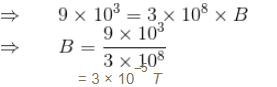The correct answer is: 3.0 × 10–5 T

QUESTION: 7

Two conducting plates of infinite extent, one plate at z = 0 and the other at z = L, both parallel to the xy-plane. Then the vector and scalar potential in the region between the plates is given by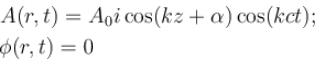For this to represent a standing wave in the empty region between the plates

Solution: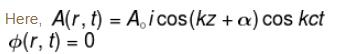For this to represent standing wave
A(z = 0) = 0 and A(z = L) = 0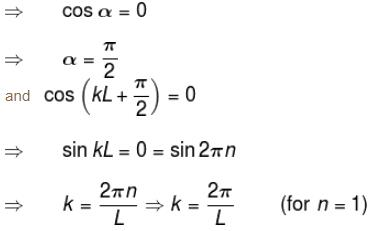The correct answer is: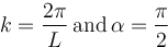QUESTION: 8

Which one of the following statements regarding the electric fields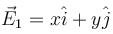and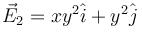is correct?

Solution:

We know that electrostatic forces are given as –ve of gradient of a potential i.e.,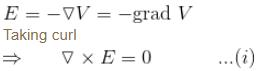(Since curl of grad is zero)
Thus, Equation. (i) implies that electrostatic fields are those, for curl E1.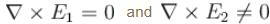E1 is electrostatic field.
The correct answer is: Only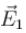can represent electrostatic field

QUESTION: 9

Consider the propagation of electromagnetic waves in a linear, homogeneous and isotropic material medium with electric permittivity ε, and magnetic permeability µ. Then for a plane wave of angular frequency ω and propagation vector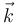propagating in the medium, Maxwell’s equations reduce to

Solution: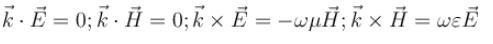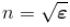The correct answer is: